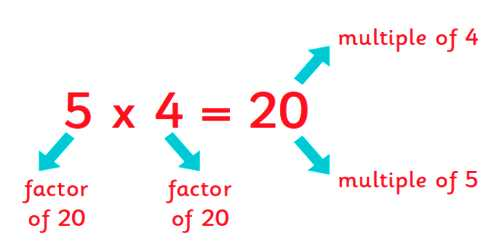Factors in mathematics, a number or algebraic expression that divides another number or expression evenly-i.e., with no remainder. For example, 3 and 6 are factors of 12 because 12 ÷ 3 = 4 exactly and 12 ÷ 6 = 2 exactly. A number may be made by multiplying two or more other numbers together. The numbers that are multiplied together are called factors of the final number. All numbers have a factor of one since one multiplied by any number equals that number. All numbers can be divided by themselves to produce the number one. Therefore, we normally ignore one and the number itself as useful factors.

In the problem, 3 x 4 = 12, 3 and 4 are factors and 12 is the product.

Factoring is like taking a number apart. It means to express a number as the product of its factors. Factors are either composite numbers or prime numbers (except that 0 and 1 are neither prime nor composite).

The number 12 is a multiple of 3 because it can be divided evenly by 3.

3 x 4 = 12

3 and 4 are both factors of 12

12 is a multiple of both 3 and 4.The number fifteen can be divided into two factors which are three and five.

The number twelve could be divided into two factors which are 6 and 2. Six could be divided into two further factors of 2 and 3. Therefore the factors of twelve are 2, 2, and 3.

If twelve was first divided into the factors 3 and 4, the four could be divided into factors of 2 and 2. Therefore the factors of twelve are still 2, 2, and 3.

A factor is simply a number that is multiplied to get a product. Factoring a number means taking the number apart to find its factors–it’s like multiplying in reverse. Here are lists of all the factors of 16, 20, and 45.

• 16 –> 1, 2, 4, 8, 16
• 20 –> 1, 2, 4, 5, 10, 20
• 45 –> 1, 3, 5, 9, 15, 45

and,

• 12 –> 12, 24, 36, 48, 60, . . .
• 5 –> 5, 10, 15, 20, 25, . . .
• 7 –> 7, 14, 21, 28, 35, . .

There are several clues to help determine factors.

• Any even number has a factor of two
• Any number ending in 5 has a factor of five
• Any number above 0 that ends with 0 (such as 10, 30, 1200) has factors of two and five.

To determine factors see if one of the above rules apply (ends in 5, 0 or an even number). If none of the rules apply, there still may be factors of 3 or 7 or some other number.

Factors are either composite numbers or prime numbers. A prime number has only two factors, one and itself, so it cannot be divided evenly by any other numbers. Here’s a list of prime numbers up to 100. You can see that none of these numbers can be factored any further.

Information Source: